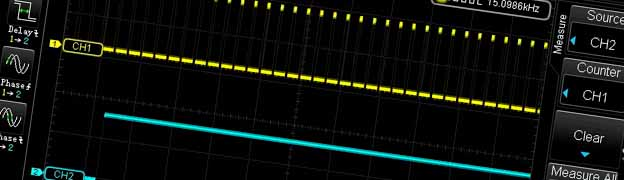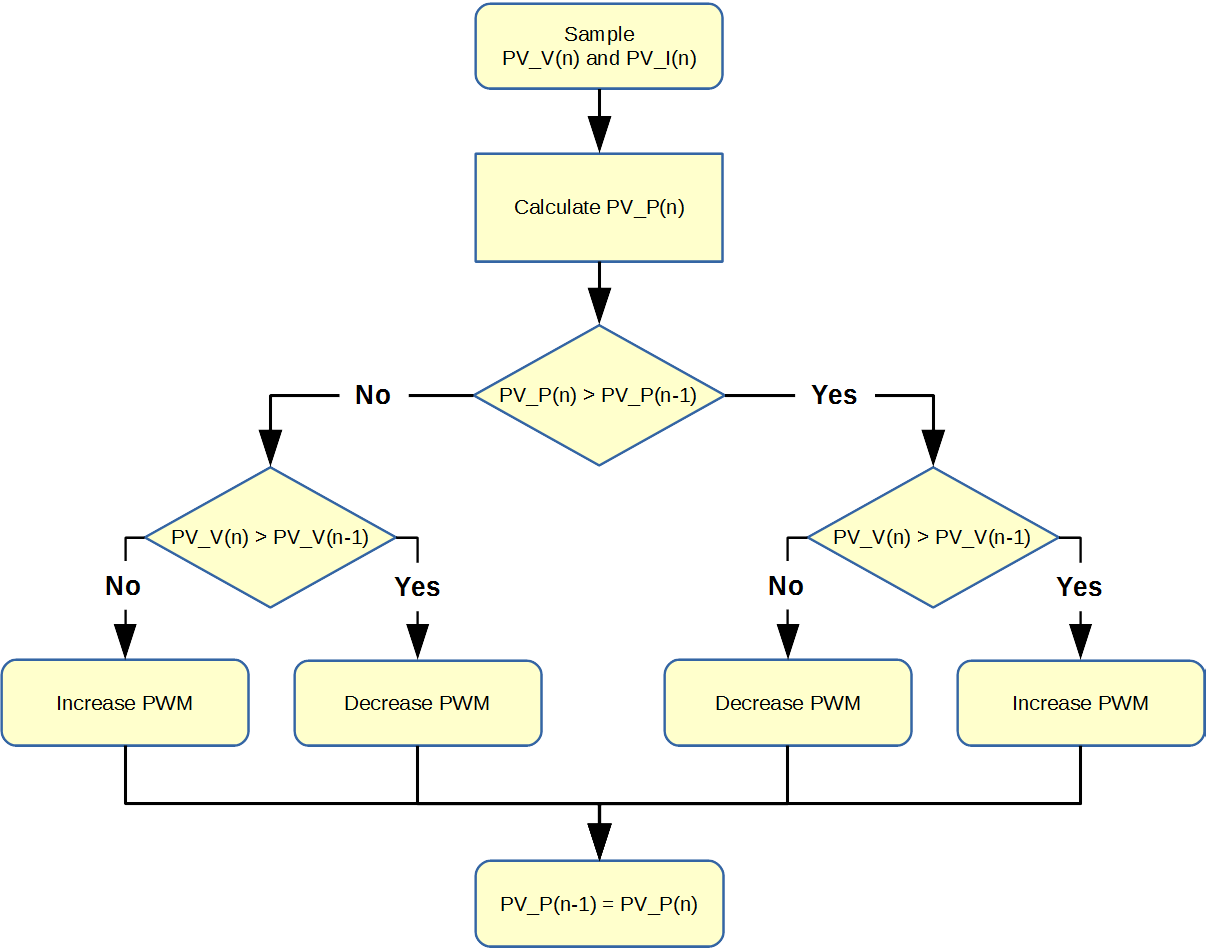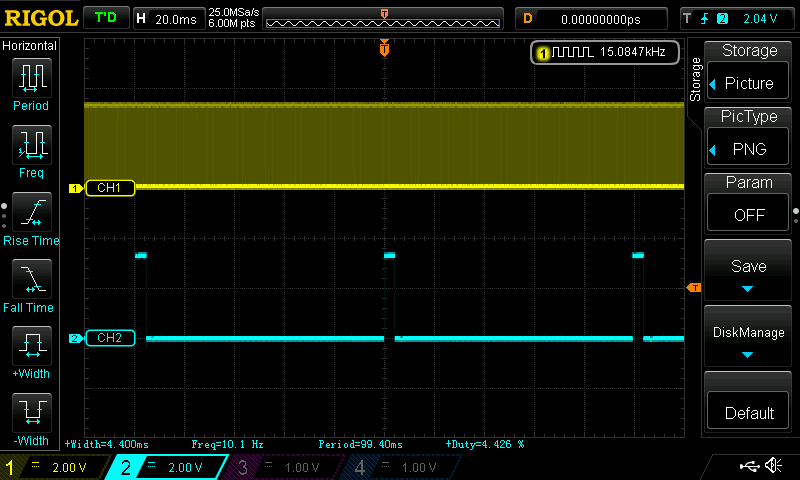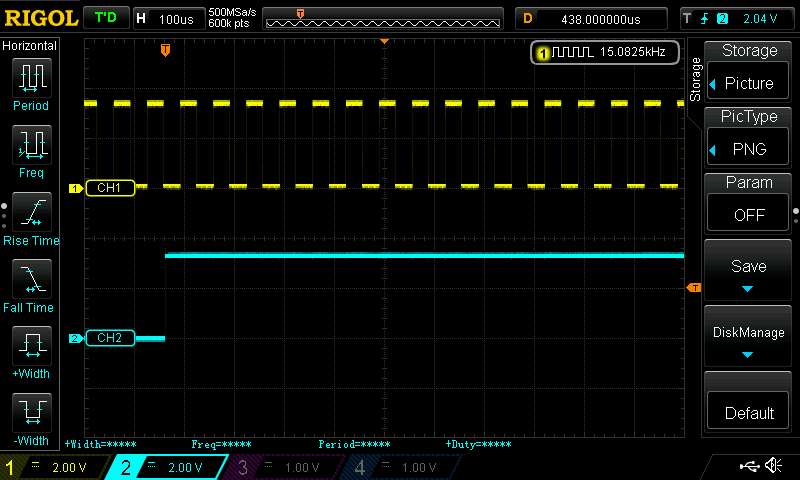# C2000 Solar MPPT tutorial Pt/3

In this third part of the C2000 Solar MPPT Tutorial, the software will be looked at in greater detail.  This will entail a look at the Perturb & Observe algorithm, ADC code and timing code to ensure everything operates in a controlled manner.

The software is based around a Perturb and Observe (P&O) algorithm, the P&O algorithm falls under the category of a hill climbing algorithm.  Hill climbing algorithms are named so due to the algorithm taking steps over sampled data to reach a desired value, in the case of the P&O this takes steps towards the MPP by increasing or decreasing the duty cycle.  There are other hill climbing algorithms such as dP/dV Feedback Control and Incremental Conductance, I intend to revisit these and write code at a later date, but for now will focus on the P&O.  Some further information on MPPT algorithms can also be found here.

## Perturb & Observe Algorithm

The P&O algorithm is a relatively simple algorithm, as such it has a few drawbacks:

• The algorithm can be confused and track in the wrong direction, this can occur under fast changing irradiance conditions, the severity of this confusion depends on the P&O setup i.e. step size and update frequency.
• The algorithm oscillates around the set point showing characteristics of an on/off controller.  More on this can be found on a previous tutorial I wrote regarding PID control, which can be found here.

The flowchart below shows the P&O algorithm used for this projectAs can be seen from the flowchart the algorithm is fairly easy to follow, turning this into C code is also relatively easy, the final C code P&O algorithm can be seen below inside the function Adj_PWM().

```void Adj_PWM(void) {
int PWM_Temp;
PWM_Temp = EPwm1Regs.CMPA.half.CMPA;

if (New_PW_In > Old_PW_In) {
if (IP_Volt > Old_IP_Volt)
{
PWM_Temp += 2;
}
else
{
PWM_Temp -= 2;
}
}
else {
if (IP_Volt > Old_IP_Volt)
{
PWM_Temp -= 2;
}
else
{
PWM_Temp += 2;
}
}

if (PWM_Temp < 100) {
PWM_Temp = 100;
}
if (PWM_Temp > 900) {
PWM_Temp = 900;
}
EPwm1Regs.CMPA.half.CMPA = PWM_Temp;
EPwm2Regs.CMPA.half.CMPA = PWM_Temp;

Old_IP_Volt = IP_Volt;
Old_PW_In = New_PW_In;
}```

So lets now run through the code briefly starting with line 3, this basically assigns the value in the counter compare A register to variable PWM_Temp.  PWM_Temp could simply be assigned to a temporary global variable, but I chose to get it straight from the register in this case.  Lines 5 to 24 form the main body of the algorithm, looking back at the flowchart the first two steps “Sample” and “Calculate” are carried out elsewhere in the ADC section of the code.  Lines 5 to 14 are illustrated by the right hand branch of the flowchart and lines 15 to 24 are illustrated by the left hand branch of the flowchart.

You have some simple if and else statements that determine which direction the algorithm takes, which is dependant on the sampled ADC data.  The result of these steps will either increase or decrease the PWM duty cycle, this increase or decrease determines the step size and in this case that value is 2.

The next block of code from lines 26 to 31 are used to prevent the duty cycle from reaching too large, and too small a value.  This was used during tuning, but also serves to provide some boundaries for the PWM, for example the duty cycle for the half bridge MOSFET drivers cannot exceed 99%, or the boost function will not operate correctly.

Lines 32 and 33 are used to update the duty cycle to the counter compare A registers for PWM1 and PWM2, both are the same duty cycle but PWM2 is 180o out of phase with PWM1.  Line 35 then assign the latest calculated solar panel voltage IP_Volt to the variable Old_IP_Volt and line 36 assign the latest calculated solar panel power New_PW_In to the variable Old_PW_In, both these variables are then used when the Adj_PWM() function is called again.

In order to help visualise the two PWM signals, the below image shows an oscilloscope trace with PWM1 in yellow and PWM2 in blue, both are set to 50% duty cycle and PWM2 is out of phase by 180o with PWM1.The next piece of code to be looked at is the ADC, I am not going to show the set-up code for the ADC or the PWM that triggers the ADC SOC, but will just show the code relating to the sampling and calculation.  However I intend to write a tutorial on each peripheral inside the C2000 with code examples, when time allows.  The ADC sampling is triggered by the PWM on every first event, therefore the sampling rate is 15ksps.  The final ADC sampling code can be seen below inside the function Data_Update()

```void Data_Update(void)
{
int numberOfSamples = 64;
int i = 0;

for(i=0; i<numberOfSamples; i++)
{

}

New_PW_In = (IP_Volt * IP_Amp);
New_PW_Out = (OP_Vol t * OP_Amp);
}```

So starting with lines 3 to 6 these are the local variables used for the function, the two floats are used to store the ADC values and the two integers are used to determine how many samples in the for loop.  The float in line 4 is a float array with four arrays, now the same result could be achieved with four separate floats.  I have left it as a float array for now, but if four floats were used the code should be optimised, by using 64 samples the following division of 128 (lines 23 to 26) could be substituted with a right bit wise shift of 7.

Lines 23 to 26 divide the sum_of_ADC_samples_Array[n] by 128 and assign the value to ADC_An floats.  Lines 28 to 31 convert the new floats to real world voltages read on the GPIO. Lines 33 to 36 then use constant values calculated from the electronic component values in the circuitry, to convert the floats to actual voltages and currents sampled in the circuit.  Lines 38 to 40 simply convert the input voltage and current to an input power and the output voltage and current to an output power.

## Timing Code

The timing code is quite critical as it determines the update frequency of the MPPT, it must also ensure the code does not overrun and cause unpredictable behaviour.  The internal timer module was used, Timer 0 was set-up to trigger an interrupt every 100mS or 10Hz. The interrupt code is shown below.

```interrupt void cpu_timer0_isr(void)
{
SysTick = 1;
PIE_clearInt(myPie, PIE_GroupNumber_1);
}```

When the interrupt is called an integer called SysTick is set to one, then the interrupt flag is cleared allowing the interrupt request to be executed and exited quickly.

Inside the main function there is a continuous while loop, the following code is run inside this loop.

```    while(1)
{
if (SysTick == 1)
{
GpioDataRegs.GPASET.bit.GPIO19 = 1;

Data_Update();

GpioDataRegs.GPACLEAR.bit.GPIO19 = 1;

SysTick = 0;
}
}```

Every time the interrupt sets the integer called SysTick to one, it allows the functions Data_Update() and Adj_PWM() to be executed, once these functions have completed SysTick is set to zero.  There are some additional lines of code on line 5 and line 10, these are used for testing and allowing the code execution time to be displayed on an oscilloscope.  The code on line 5 switches GPIO pin 19 high, then the code on line 10 switches GPIO pin 19 back to low, so a square wave pulse is produced and the pulse width gives an indication of the code execution time of the functions Data_Update() and Adj_PWM(). The following images show captures from an oscilloscope.This first image shows the 15kHz PWM being displayed on channel 1, the individual wave pulses are not visible as the time base is set to display channel 2.  The blue trace shown on channel 2 can be seen to have a frequency of 10Hz, with a pulse width of 4.4mS, so the functions Data_Update() and Adj_PWM() take 4.4mS to execute.  Putting this into context there should be 128 ADC samples captured, taken from 64 triggers of the PWM signal, therefore 64*66.67uS (one 15kHz cycle) = 4.27mS.  A single ADC sample and conversion takes around 650nS, if we multiply that by the 8 samples, a conversion is being completed every 8*650nS = 5.2uS (it will be faster than this due to ADC pipelining effects).  It can be clearly seen that there is plenty of room for more oversampling if required, as the 5.2uS sample and conversion time easily fits inside the 66.67uS window of the PWM trigger.  There is also a small amount of code overhead being added artificially by toggling GPIO pin 19, which is not significant but something to be aware of.The second image has a smaller time base setting (100uS) effectively zooming in, which allows the individual pulses from the 15kHz PWM to be visible.  So going back to the step size of 2 shown in the Adj_PWM() function, this can be put into context when the maximum duty cycle value as a variable, for 100% duty cycle equals 1000.  Therefore with a PWM update frequency of 10Hz and a maximum step size of 2, this equates to a maximum duty cycle change of 2% per second.

I captured some video which shows 3 variables being graphed in Code Composer Studio, these variables are PV Power, PV Volts and the Duty Cycle.  The video also demonstrates the MPPT in action under simulated fast changing cloud conditions, as well as some natural cloud.

There will be one final part to this series of tutorials this will cover some of the set-up and testing, and will also include a link to the full C code for the project.

## 11 thoughts on “C2000 Solar MPPT tutorial Pt/3”

1.Peter Michael says:

Hi Ant, thanks for sharing this project, it is very useful.
Why do you calculate the “New_PW_Out”, where we need to use that? thanks

1.Ant says:

Hi Peter,

This is probably 2 years too late this reply, this was calculated so the losses in the circuit could be determined

2.aditya says:

How i can implement P&O algorithm and battery charging algorithms simultaneously using msp430g2553?

1.Ant says:

Hi,

The MPPT algorithm will track the solar panel changing power, but the output of the MPPT will not be a regulated voltage. So a further step is to have another SMPS topology to regulate the supply to your needs. A PID algorithm maybe a solution and then you need to bring the 2 algorithms together and ensure the update frequency of both meets your needs.

Cheers,
Ant

3.Karan Patil says:

Can you post the details of ADC configuration?
I am using C2000 Piccolo launchpad for sampling 6 ADC channels triggered by epwm1 with frequency of 20kHz. However sampling frequency is roughly about 4kHz. I had posted on TI forum but problem didnt get solved.

1.Ant says:

Hi Karan,

Regards,
Ant

1.Peter Michael says:

Hi Ant,

Congratulations for tour project.

Thanks

1.Ant says:

I have now removed all Adfly links so everything is directly downloaded from the Coder-tronics website, sorry this has taken so long but been pretty busy

4.jinkai says:

I have a question. The P&O flowchart seems to be at odds with your graph in the YouTube video:

At the start of the graph,
1) PV_P(n) > PV_P(n-1)
2) PV_V(n) > PV_V(n-1)
3) Hence duty cycle is decreased.

However, (3) disagrees with the flowchart, which states that PWM should increase (the rightmost “increase PWM” block).

Did I misinterpret something?

1.Ant says:

Hi Jinkai,

Nope no misinterpretation and well spotted, but think about the electronics. The MOSFET driver is a non-inverting type, however the MOSFETs invert the output and produce the output you see in the video.

Cheers,
Ant